# Tour of Britain

The Tour of Britain is coming to our local roads in Dorset and the Isle of Wight. This is, most likely, a once in a lifetime opportunity to watch the pro cyclists on the roads we ride week in, week out, all year round.

Our exciting local stages will be:

• Stage seven | Saturday 10 September | West Bay to Ferndown
• Stage eight | Sunday 11 September | Ryde to The Needles

As part of the celebration, I’ve rendered the full 2022 Tour of Britain route… The final animation:

• has over 66,000 objects and over 30,000,000 vertices
• took up to 15mins per frame for 5,760 frames = over 30 days rendering

Stage 1 - Aberdeen to Glenshee Ski Centre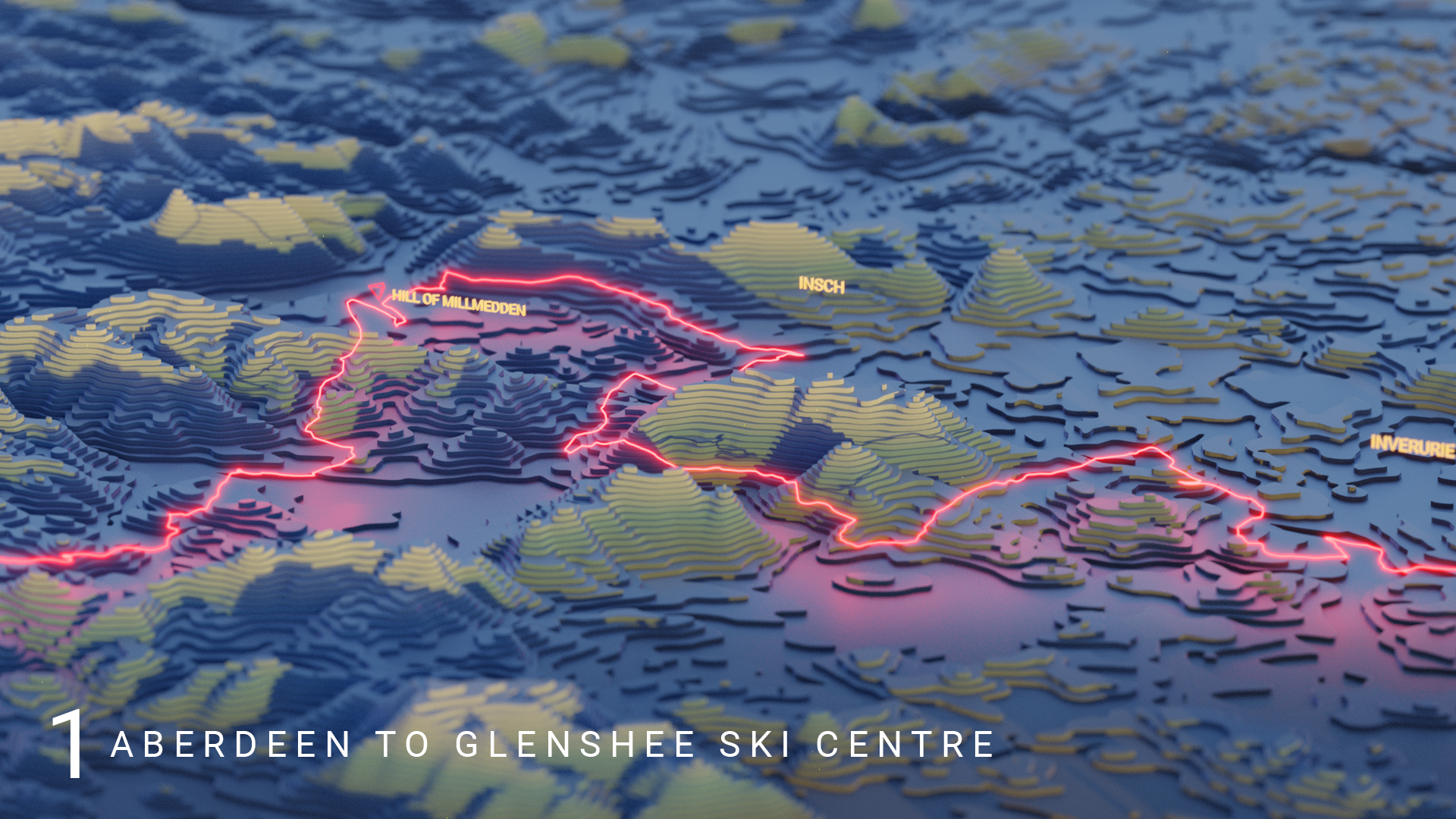Stage 2 - Hawick to DunsStage 3 - Durham to Sunderland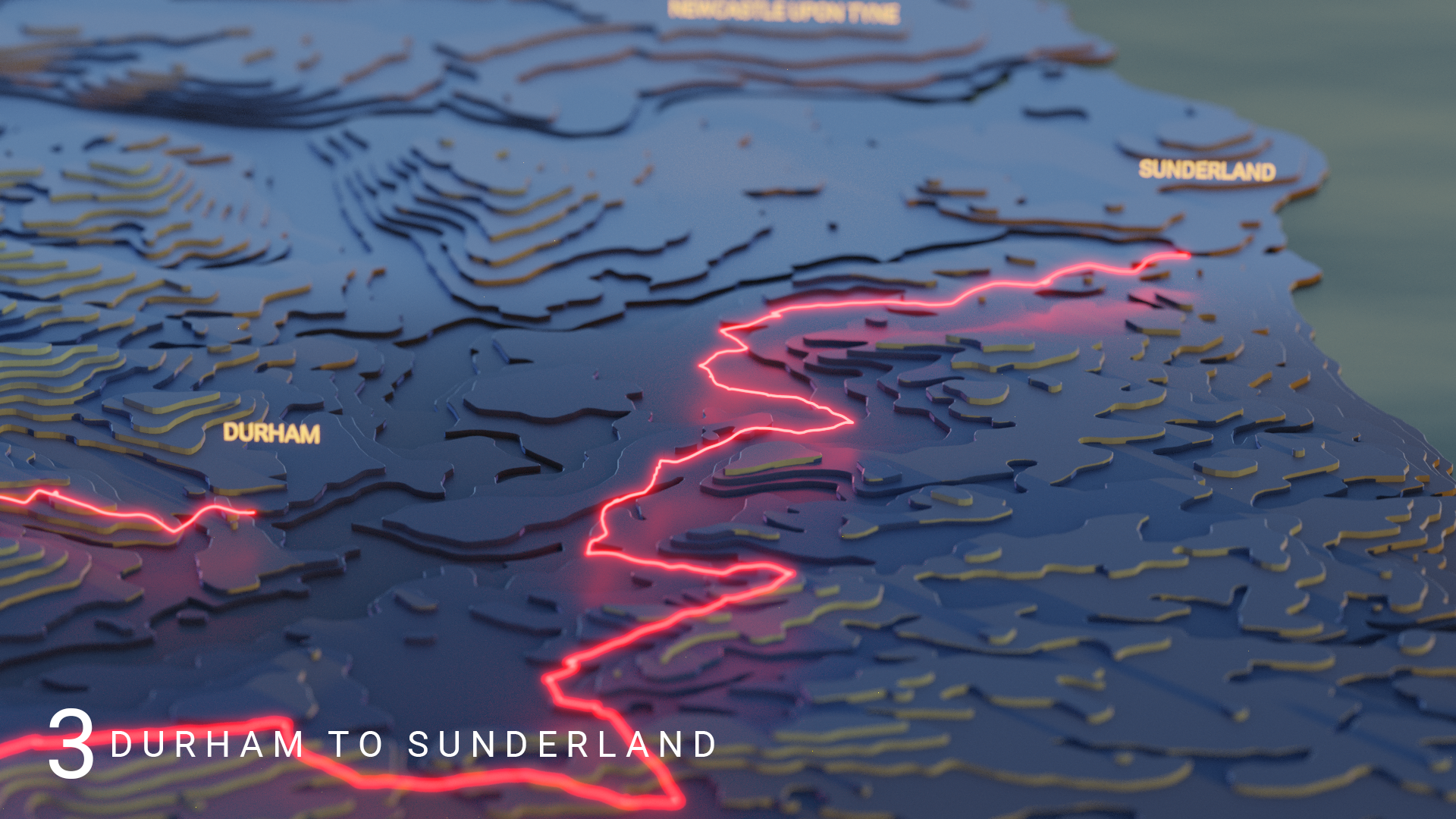Stage 4 - Redcar to Duncombe Park, Helmsley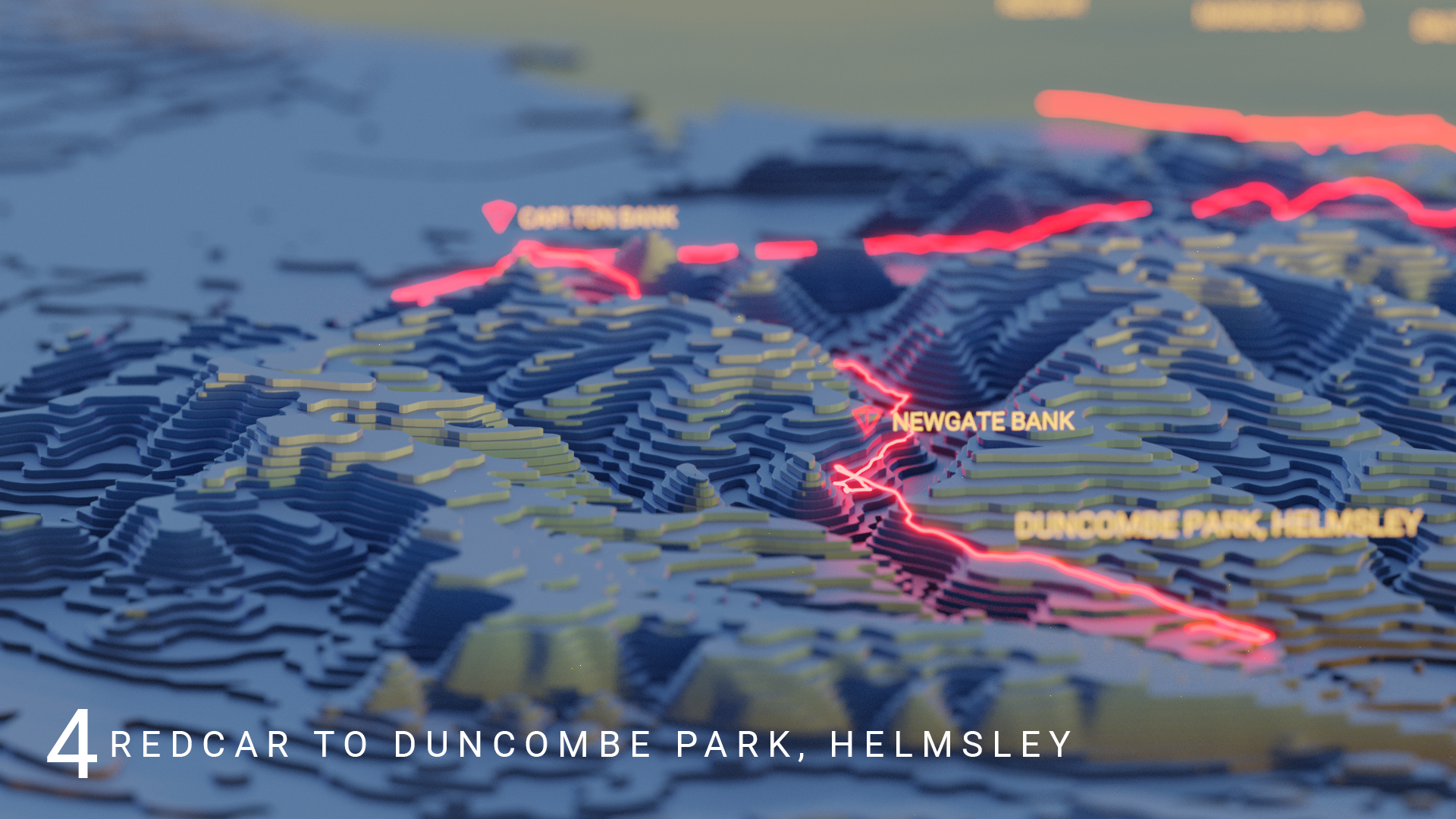Stage 5 - West Bridgford to MansfieldStage 6 - Tewkesbury to GloucesterStage 7 - West Bay to Ferndown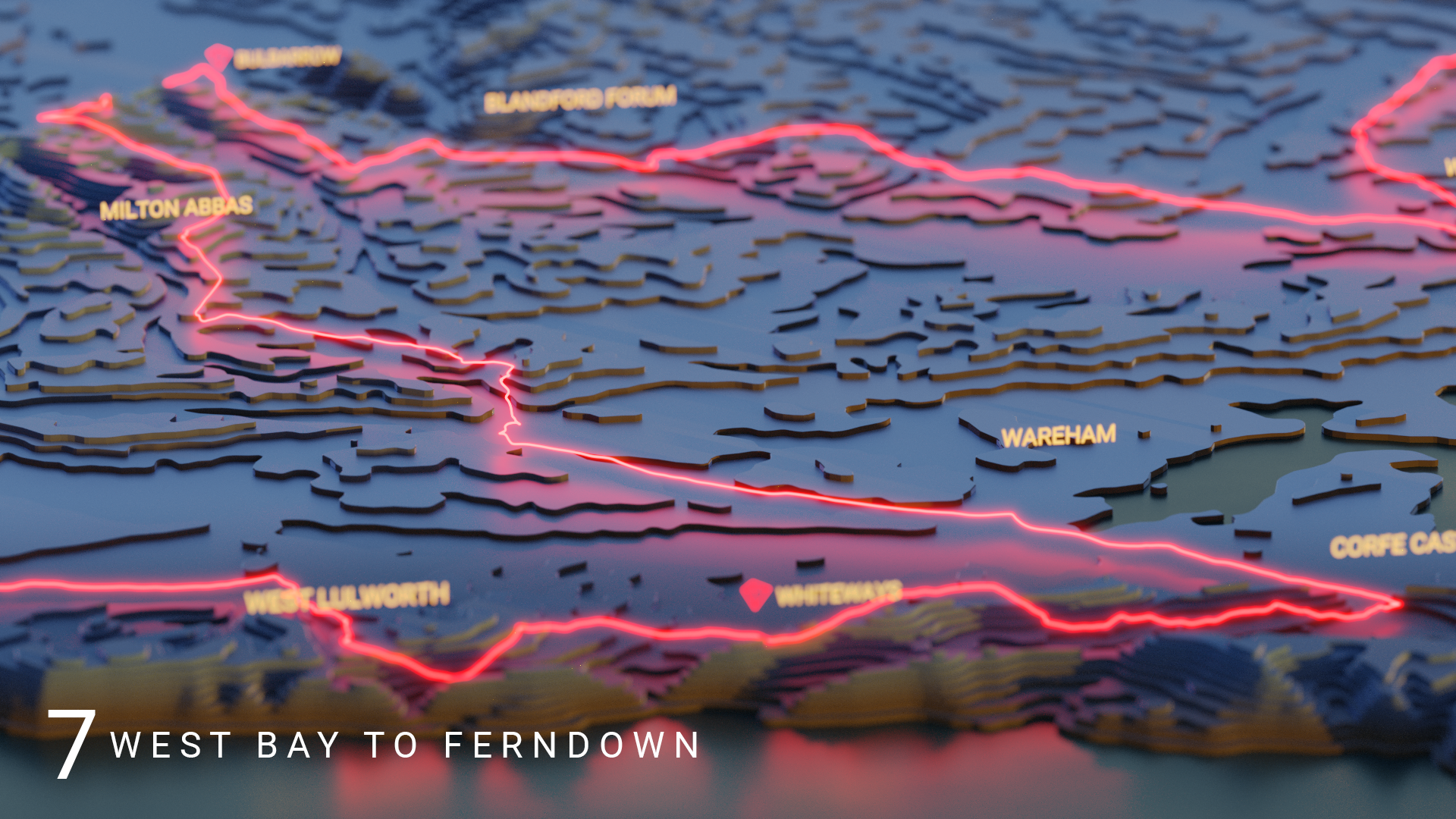Stage 8 - Ryde to The Needles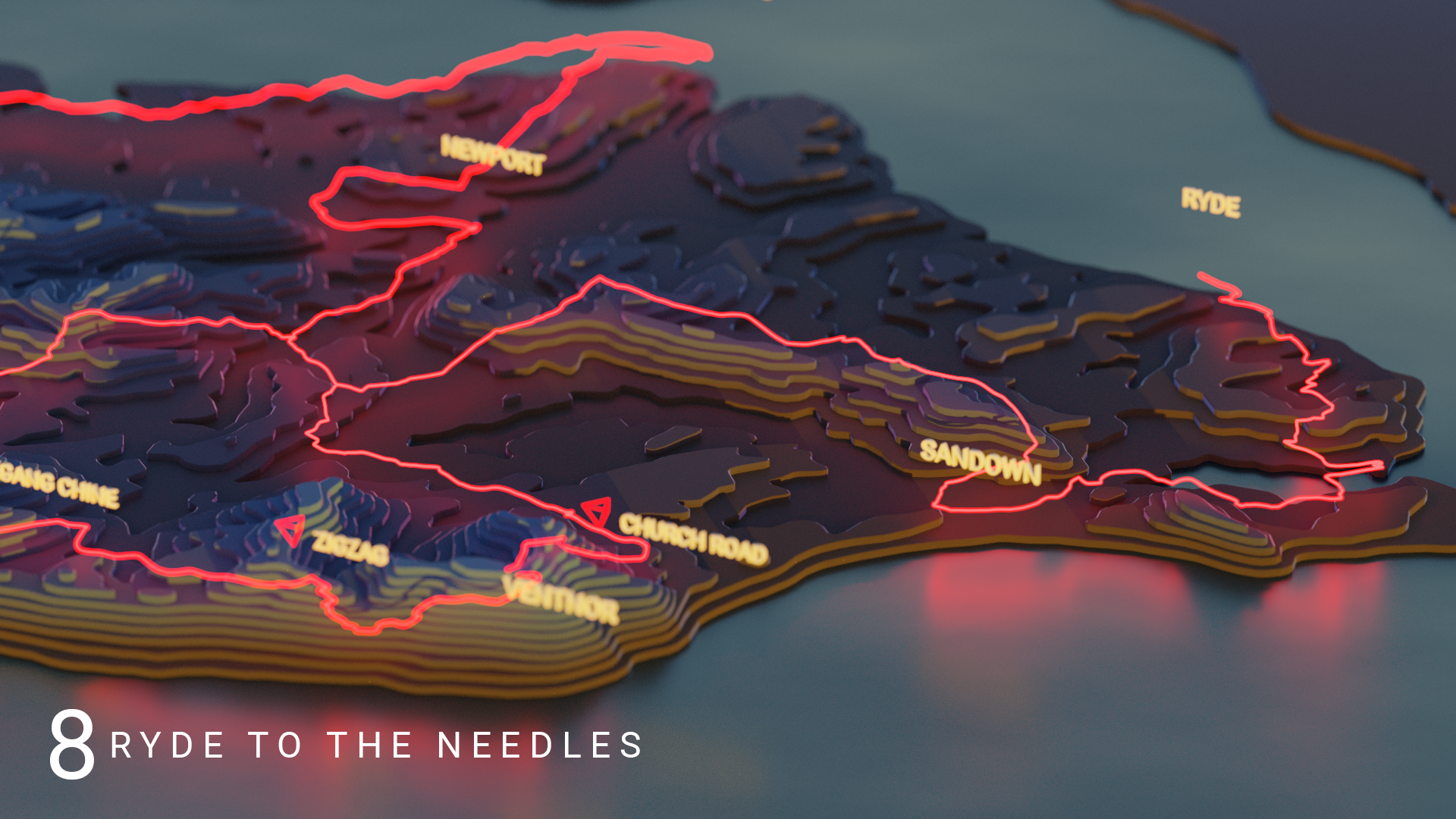Behind the Scenes

I often refer to these animations as an Agile journey - primarily because they are an incremental exploration of a concept over time. People can get caught up with the Agile Frameworks such as Scrum, Kanban, XP etc but fundamentally, it’s a mindset rather than a method: Experiment, Evolve, Improve. As part of this behind-the-scenes piece, I wanted to try and capture this…

The Tour of Britain (ToB) concept started as:

I’ve already mapped the Purbecks, I’ll extend to the rest of the route.

This in turn translated into a rough initial todo list (backlog):

• create UK elevation model (3D Tanaka contours)
• choose materials and lighting
• model one stage and animate
• choose music
• repeat for remaining stages
• create final video

It ended up with a fair few more items…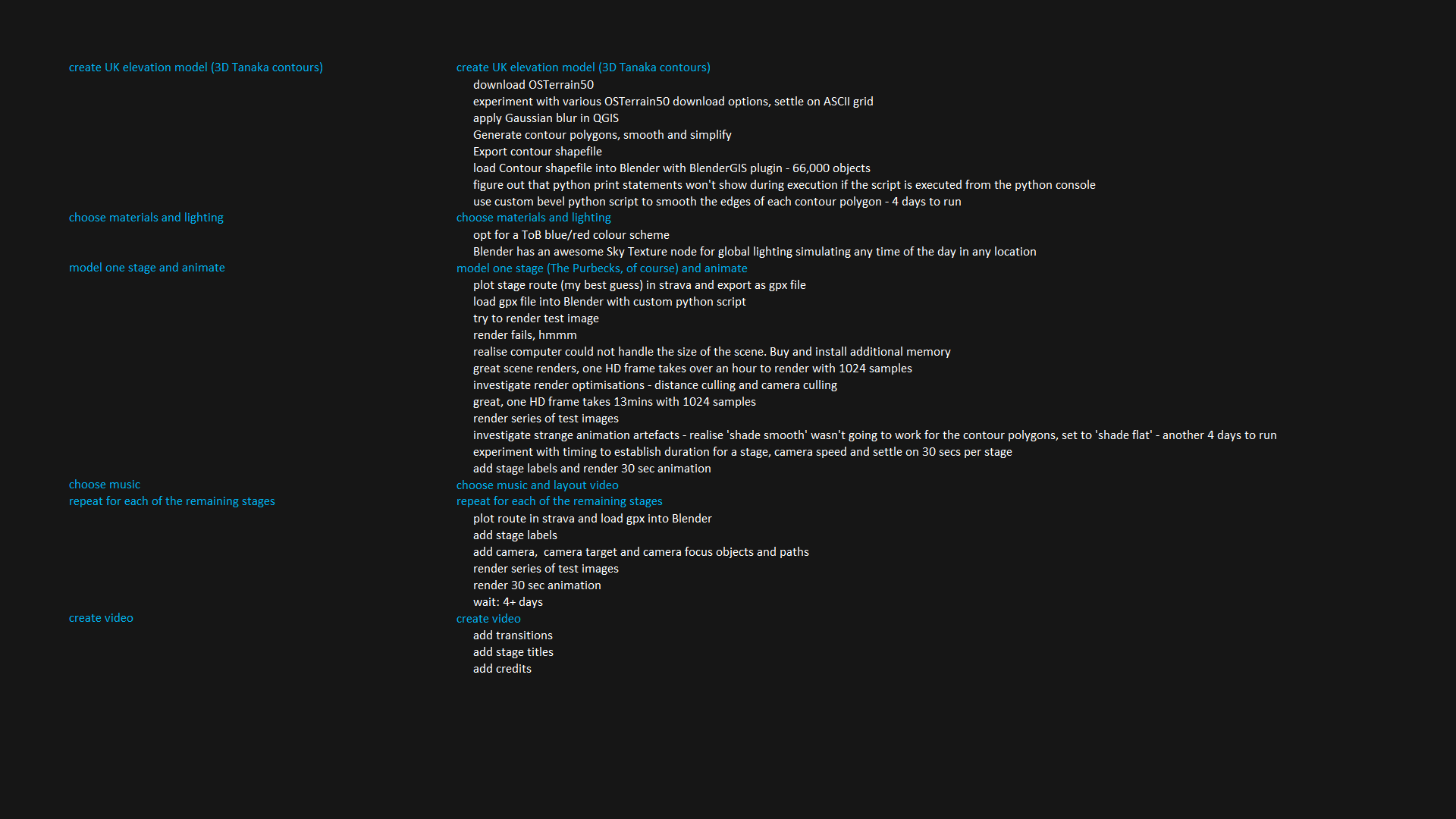There was a fair amount of preparation to create the UK contour model and the first stage, but from that point onwards, modelling progress was pretty quick. Rendering less so - being unable to render the scene after waiting the best part of a week to create the poloygons was a definite low point. Fortunately some more memory and some rendering optimisations solved that issue but even then, taking between 5 and 15 minutes per frame, a stage was takes between 4 and 7 days to render. One decision I did make that worked out well was to model the gpx tracks, stage labels and camera paths in a separate blend file linking into to the master. This meant I could continue modelling and editing while the lengthy render process was running. A further refinement of this would be to render the labels as a separe ViewLayer which (I think) would mean the labels could be updated without needing to re-render the entire animation. One to investigate…

Custom Bevel Script

For some reason I couldn’t get the Bevel Modifier to work with the imported shapefiles. I suspect it’s because they are either an n-gon or a low density triangular mesh. I experimented with creating my own bevel script and it turned out pretty well. The concept was straightward - approximate the tangent/normal at a vertex using the immediate vertices on either side and then use trigonometry against the normal to shrink the shape inwards: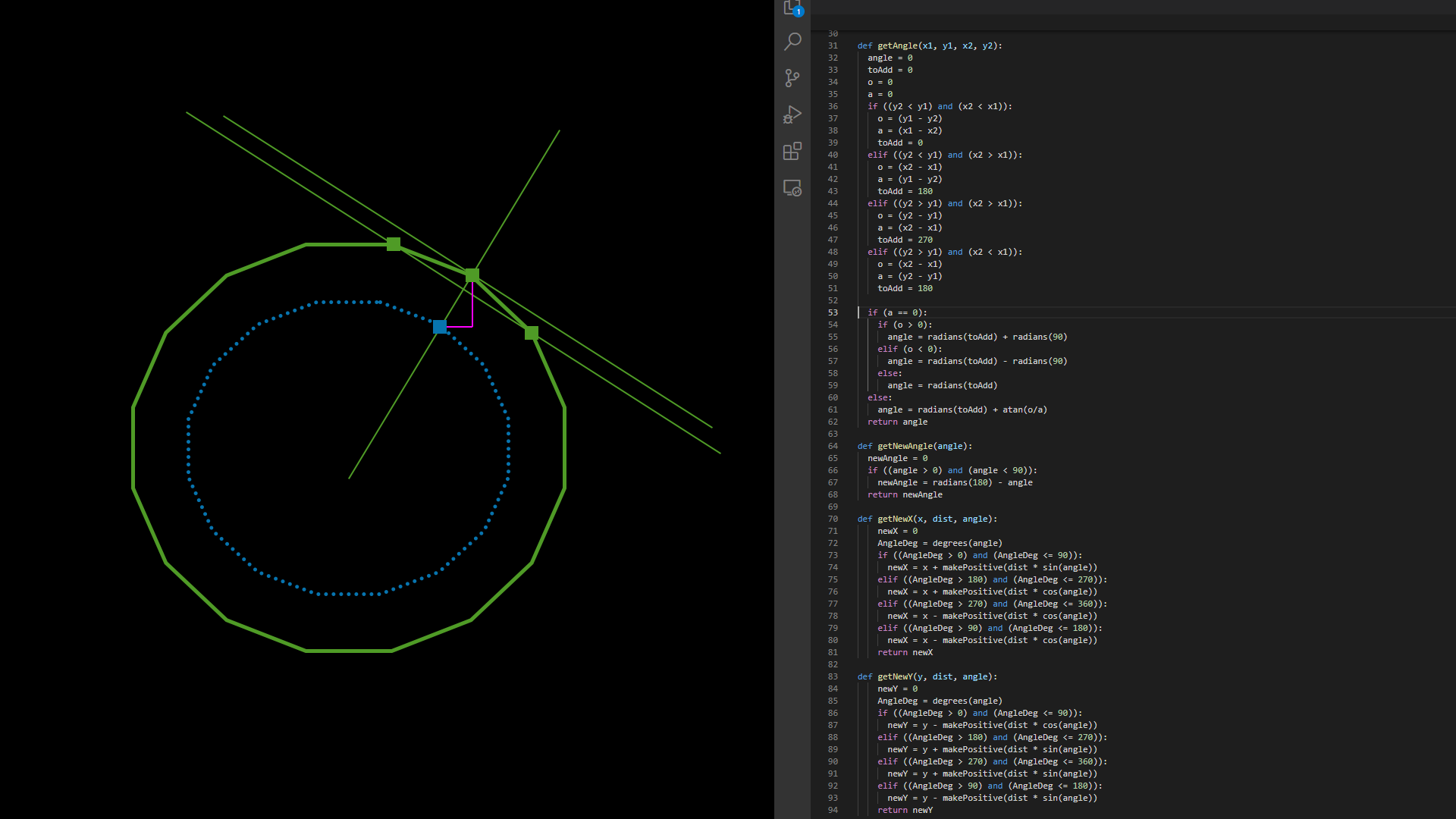Coupling together a ShrinkObject() function with Blender’s extrude function worked pretty well!… Now that I’m looking again at the script, it’s only using the current and next vertices, rather than previous and next, so room for an improved version two!!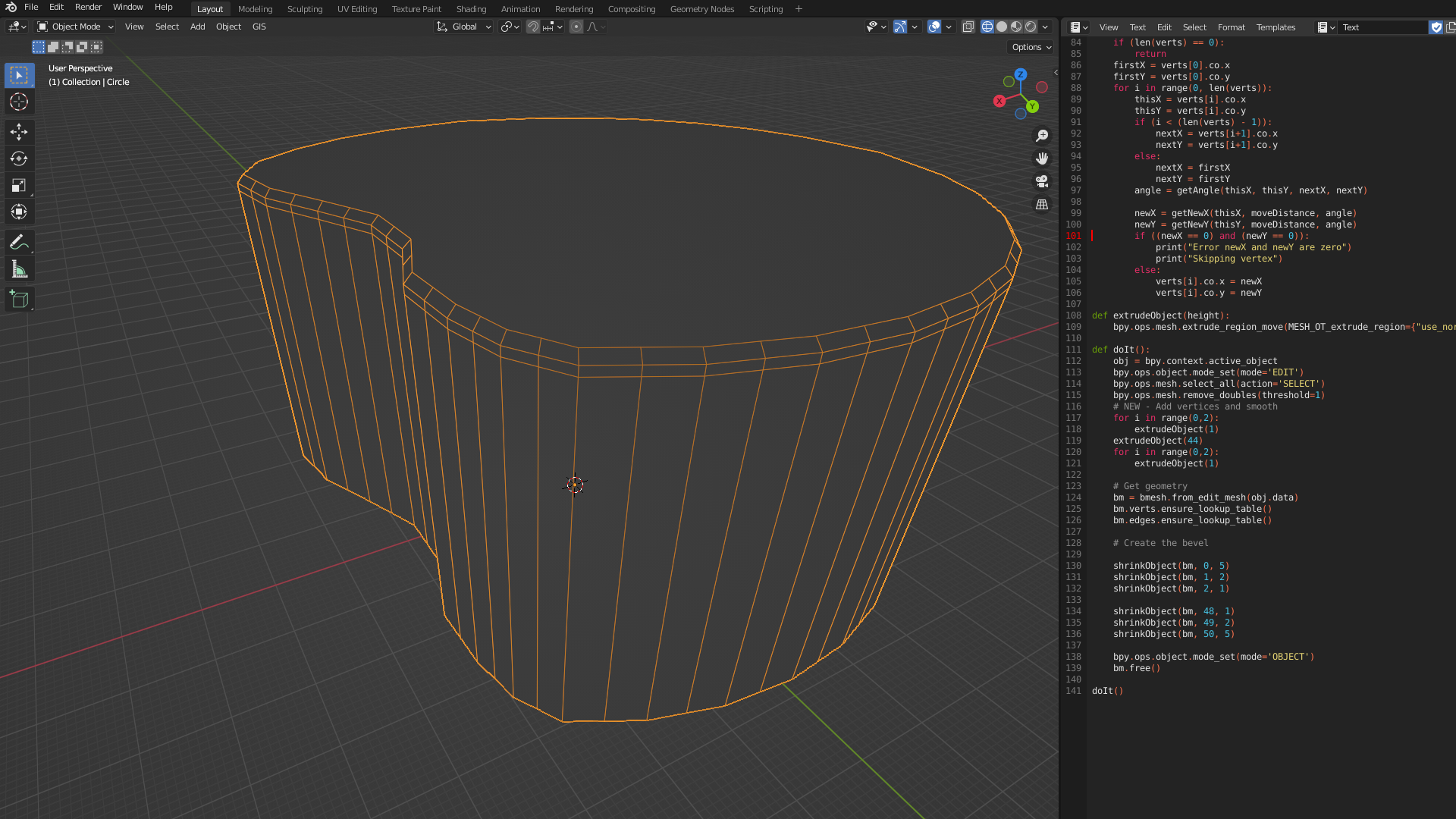In case anyone is interested, here’s the code:

``````# Custom bevel script. Run on imported contour polygon mesh.

import bpy, bmesh
from math import atan, cos, degrees, isclose, radians, sin, sqrt
from mathutils import Vector
from bmesh.types import BMVert
import sys

def writeToLogFile(msg):
filename = 'c://temp//blenderScriptLog.txt'
with open(filename, 'a') as f:
f.write(msg)
f.write('\n')
print(msg)

def makePositive(n):
return (sqrt(n * n))

def getAngle(x1, y1, x2, y2):
angle = 0
o = 0
a = 0
if ((y2 < y1) and (x2 < x1)):
o = (y1 - y2)
a = (x1 - x2)
elif ((y2 < y1) and (x2 > x1)):
o = (x2 - x1)
a = (y1 - y2)
elif ((y2 > y1) and (x2 > x1)):
o = (y2 - y1)
a = (x2 - x1)
elif ((y2 > y1) and (x2 < x1)):
o = (x2 - x1)
a = (y2 - y1)

if (a == 0):
if (o > 0):
elif (o < 0):
else:
else:
return angle

def getNewAngle(angle):
newAngle = 0
if ((angle > 0) and (angle < 90)):
return newAngle

def getNewX(x, dist, angle):
newX = 0
AngleDeg = degrees(angle)
if ((AngleDeg > 0) and (AngleDeg <= 90)):
newX = x + makePositive(dist * sin(angle))
elif ((AngleDeg > 180) and (AngleDeg <= 270)):
newX = x + makePositive(dist * cos(angle))
elif ((AngleDeg > 270) and (AngleDeg <= 360)):
newX = x - makePositive(dist * cos(angle))
elif ((AngleDeg > 90) and (AngleDeg <= 180)):
newX = x - makePositive(dist * cos(angle))
return newX

def getNewY(y, dist, angle):
newY = 0
AngleDeg = degrees(angle)
if ((AngleDeg > 0) and (AngleDeg <= 90)):
newY = y - makePositive(dist * cos(angle))
elif ((AngleDeg > 180) and (AngleDeg <= 270)):
newY = y + makePositive(dist * sin(angle))
elif ((AngleDeg > 270) and (AngleDeg <= 360)):
newY = y + makePositive(dist * sin(angle))
elif ((AngleDeg > 90) and (AngleDeg <= 180)):
newY = y - makePositive(dist * sin(angle))
return newY

def shrinkObject(bm, z, moveDistance):

verts = []
for v in bm.verts:
#print("v: %f, %f, %f" % (v.co.x, v.co.y, v.co.z))
if isclose(v.co.z, z, rel_tol=0.01):
verts.append(v)
writeToLogFile("found %d vertices at height %f" % (len(verts), z))
if (len(verts) == 0):
return
# print("a: %f %f" % (a, a * 180 / pi))
# Save the co-ords of the first point otherwise it will have already moved when we get there
firstX = verts.co.x
firstY = verts.co.y
#for i in range(0, 26):
for i in range(0, len(verts)):
#print("i: %d" % i)
thisX = verts[i].co.x
thisY = verts[i].co.y
if (i < (len(verts) - 1)):
nextX = verts[i+1].co.x
nextY = verts[i+1].co.y
else:
#print("using first point")
nextX = firstX
nextY = firstY
#print("Current: %f, %f" % (thisX, thisY))
#print("Next: %f, %f" % (nextX, nextY))
angle = getAngle(thisX, thisY, nextX, nextY)

#print("Angle: %f (degrees: %f)" % (angle, degrees(angle)))
newX = getNewX(thisX, moveDistance, angle)
newY = getNewY(thisY, moveDistance, angle)
#print("New: %f, %f" % (newX, newY))
if ((newX == 0) and (newY == 0)):
print("Error newX and newY are zero")
print("Skipping vertex")
else:
verts[i].co.x = newX
verts[i].co.y = newY

def extrudeObject(height):
bpy.ops.mesh.extrude_region_move(MESH_OT_extrude_region={"use_normal_flip":False, "use_dissolve_ortho_edges":False, "mirror":False}, TRANSFORM_OT_translate={"value":(0, 0, height), "orient_type":'GLOBAL', "orient_matrix":((1, 0, 0), (0, 1, 0), (0, 0, 1)), "orient_matrix_type":'GLOBAL', "constraint_axis":(False, False, True), "mirror":False, "use_proportional_edit":False, "proportional_edit_falloff":'SMOOTH', "proportional_size":1, "use_proportional_connected":False, "use_proportional_projected":False, "snap":False, "snap_target":'CLOSEST', "snap_point":(0, 0, 0), "snap_align":False, "snap_normal":(0, 0, 0), "gpencil_strokes":False, "cursor_transform":False, "texture_space":False, "remove_on_cancel":False, "release_confirm":False, "use_accurate":False, "use_automerge_and_split":False})

obj = bpy.context.active_object
# mark the whole object shade smooth
bpy.ops.object.mode_set(mode='EDIT')

bpy.ops.mesh.select_all(action='SELECT')

writeToLogFile('Extruding')
for i in range(0,2):
extrudeObject(-5)
extrudeObject(-80)
for i in range(0,2):
extrudeObject(-5)

# Get geometry
bm = bmesh.from_edit_mesh(obj.data)
bm.verts.ensure_lookup_table()
bm.edges.ensure_lookup_table()

# Create the bevel
writeToLogFile('Creating bevel')

shrinkObject(bm, 0, 20)

#writeToLogFile('Marking top face flat')

writeToLogFile('Continuing bevel')
shrinkObject(bm, -5, 10)
shrinkObject(bm, -10, 5)

shrinkObject(bm, -90, 5)
shrinkObject(bm, -95, 10)
shrinkObject(bm, -100, 20)

# Put faces on the top
# and mark flat

bpy.ops.object.mode_set(mode='OBJECT')
bm.free()

i = 0
for obj in bpy.data.collections['ToProcess'].all_objects:
writeToLogFile("object number: %d" % i)
writeToLogFile("obj:%s\n" % (obj.name))
bpy.ops.object.select_all(action='DESELECT')
obj.select_set(True)
selected_object = obj
bpy.context.view_layer.objects.active = selected_object